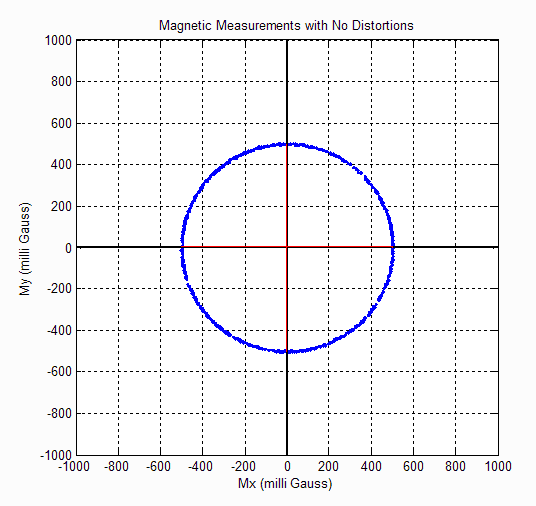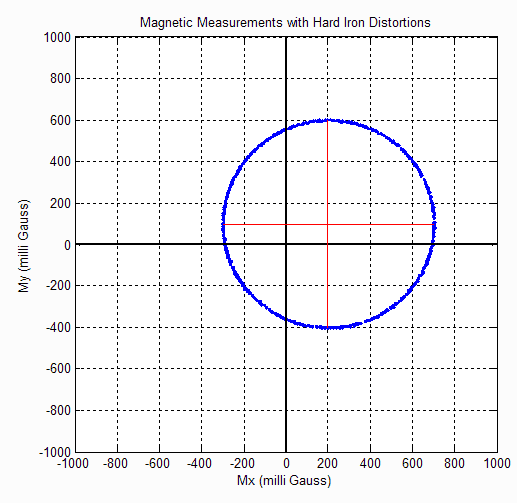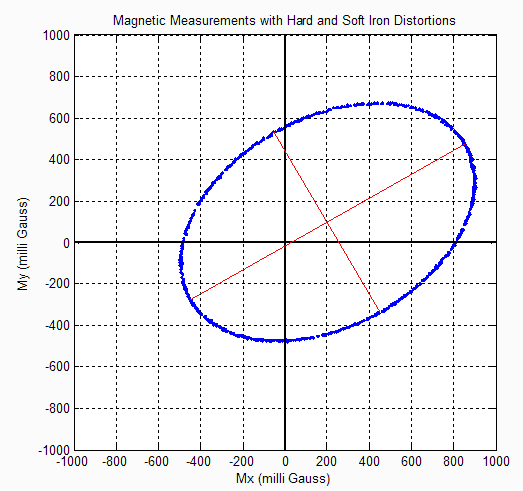# 3.5 Magnetometer Errors & Calibration

A magnetometer is a type of sensor that measures the strength and direction of the local magnetic field. The magnetic field measured will be a combination of both the earth's magnetic field and any magnetic field created by nearby objects. The magnetic field is measured in the sensor reference frame.

## Earth's Magnetic Field

The earth's magnetic field is a self sustaining magnetic field that resembles a magnetic dipole with one end near the Earth's geographic North Pole and the other near the earth's geographic South Pole. The strength of this magnetic field varies across the Earth with strengths as low as 0.3 Gauss in South America to over 0.6 Gauss in northern Canada. Although this magnetic field is relatively stable over time, electric currents in the ionosphere can cause daily alterations which can deflect surface magnetic fields by as much as one degree. Normally daily variations in field strength are on the order of 0.25 mGauss, which would equate to about a 0.03 degree variation in heading. This small of a change in heading is on the same order of magnitude as the resolution of most MEMS based magnetometers, so in most cases the Earth's magnetic field can be considered constant with respect to time.

## Magnetometer Sensor Model

A sensor model is used to mathematically correct for errors in scale factor, misalignment, and bias. The magnetometers used in VectorNav's sensors, unless otherwise stated, use a linear sensor model.

\begin{equation} H = \left[\begin{matrix} x\\y\\z\end{matrix}\right]_I = \left[\begin{matrix} 1 & M_{XY} & M_{XZ}\\ M_{YX} & 1 & M_{YZ}\\ M_{ZX} & M_{ZY} & 1\\ \end{matrix}\right] * \left[\begin{matrix} \frac{1}{S_{X}} & 0 & 0\\ 0 & \frac{1}{S_{Y}} & 0\\ 0 & 0 & \frac{1}{S_{Z}}\end{matrix}\right]\left(B_D + \left[\begin{matrix} B_X\\B_Y\\B_Z\end{matrix}\right] - \left[\begin{matrix} V_X\\V_Y\\V_Z\end{matrix}\right]\right) \end{equation} This written out as algebraic equations gives the following:

\begin{equation} H_X = \frac{B_D + B_X - V_X}{S_{X}} + \frac{M_{XY}(B_D + B_Y - V_Y)}{S_Y} + \frac{M_{XZ}(B_D + B_Z - V_Z)}{S_Z} \end{equation}

\begin{equation} H_Y = \frac{M_{YX}(B_D + B_X - V_X)}{S_{X}} + \frac{B_D + B_Y - V_Y}{S_Y} + \frac{M_{YZ}(B_D + B_Z - V_Z)}{S_Z} \end{equation}

\begin{equation} H_Z = \frac{M_{ZX}(B_D + B_X - V_X)}{S_{X}} + \frac{M_{ZY}(B_D + B_Y - V_Y)}{S_Y} + \frac{B_D + B_Z - V_Z}{S_Z} \end{equation}

## Temperature calibration

The above calibration parameters will vary with changing temperature. To account for this all VectorNav sensors utilize a third order algebraic polynomial for each calibration coefficient as a function of temperature. Each calibration coefficient can be calculated as follows:

\begin{equation} \begin{matrix} C_n = C_{n_0} + C_{n_1}\Delta T + C_{n_2}\Delta T^2 + C_{n_3}\Delta T^3 \\ where\;\Delta T = [Temperature - 25] ^{\circ} C\\ \end{matrix} \end{equation}

## Hard and Soft Iron Distortions

Magnetic measurements will be subjected to distortion. These distortions are considered to fall in one of two categories; hard or soft iron. Hard iron distortions are created by objects that produce a magnetic field. A speaker or piece of magnetized iron for example will cause a hard iron distortion. If the piece of magnetic material is physically attached to the same reference frame as the sensor, then this type of hard iron distortion will cause a permanent bias in the sensor output. Soft iron distortions are considered deflections or alterations in the existing magnetic field. These distortions will stretch or distort the magnetic field depending upon which direction the field acts relative to the sensor. This type of distortion is commonly caused by metals such as nickel and iron. In most cases hard iron distortions will have a much larger contribution to the total uncorrected error than soft iron.

## Visualizing Hard and Soft Iron Distortions

A common way of visualizing and correcting hard and soft iron distortions is to plot the output of the magnetometer on a 2D graph. The following plot shows measurements taken by the magnetometer as the device is slowly rotated around the Z-axis.

## Case 1- No Distortions

In the event that there are no hard or soft iron distortions present, the measurements should form a circle centered at X=0, Y=0. The radius of the circle equals the magnitude of the magnetic field. Hard iron distortions will cause a permanent bias to be present in the outputs. The effect of hard iron distortions on the plot will be to shift the center of the circle.Figure: 3.7 No Distortions

## Case 2 - Hard Iron Distortions

As shown in the plot the center of the circle with hard iron distortions is now at X=200, Y=100. From this we can conclude that there is 200 mGauss hard iron bias in the X-axis and 100 mGauss hard iron bias in the Y-axis. Hard iron distortions will only shift the center of the circle away from the origin. Hard iron distortions will not distort the shape of the circle. Soft iron distortions on the other hand distort and warp the existing magnetic fields. When you plot the magnetic output soft iron distortions are easy to recognize since they will distort the circular output. Soft Iron effects warp the circle into an elliptical shape. Below is an example of the magnetometer output when both hard and soft iron distortions are present.Figure: 3.8 Hard Iorn Distortions

## Case 3 - Hard and Soft Iron Distortions

As shown in the above magnetometer output, the circle has been distorted into an ellipse. The center of the ellipse is still located at X=200 mGauss and Y=100mGauss since the hard iron distortions are the same as before. Every ellipse has a major and minor axis which corresponds to the long and short dimensions respectfully. As shown in the above plot the ellipse has its major axis aligned 30 degrees up from the body frame X direction.Figure: 3.9 Hard and Soft Iorn Distortions

## How to Eliminate Hard and Soft Iron Distortions

It is possible to eliminate the effects of both hard and soft iron distortions on the magnetometer outputs. The VectorNav products use the following calibration model to correct for hard and soft iron distortions.

\begin{equation} M = \left[\begin{matrix} C_1 & C_2 & C_3\\ C_4 & C_5 & C_6\\ C_7 & C_8 & C_9\\ \end{matrix}\right] \left[\begin{matrix} H_x - C_{10}\\ H_y - C_{11}\\ H_z - C_{12}\\ \end{matrix}\right] \end{equation} The above model consists of 12 hard and soft iron compensation parameters. The first 9 parameters correct for the soft iron while the last three {C10, C11, C12} parameters compensate math_839568375 for the hard iron. In case shown in Figure 3, the hard and soft iron calibration parameters would be

\begin{equation} M = \left[\begin{matrix} .75 & -.1443 & 0\\ -.1443 & 0.9167 & 0\\ 0 & 0 & 1.0\\ \end{matrix}\right] \left[\begin{matrix} H_X - 200\\ H_Y - 100\\ H_Z - 0\\ \end{matrix}\right] \end{equation} From the above parameters you can see that the hard iron parameters are set to the offset of the center of the ellipse as described in the previous section. Also, note that the hard an soft iron effect influences all the axes of the sensor and the above example could be repeated for both rotations about the X and Y axes.